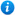# Add and Subtract Numbers Mentally - Year 3

Add and Subtract Numbers Mentally - Year 3

Year 3 maths programme of study - Number - (addition and subtraction)

Pupils should be taught to:

• add and subtract number mentally, including: 3-digit numbers and ones, 3-digit numbers and tens and 3-digit numbers and hundreds
In this maths teaching resource, pupils practise adding and subtracting numbers mentally, including: a 3-digit number and ones; a 3-digit number and tens; a 3-digit number and hundreds as per the curriculum objectives of the year 3 maths programme of study (Number - addition and subtraction).   Content includes:

• Animated PowerPoint lesson with class activities and 1 accompanying worksheet
• 3 differentiated worksheets with answers

'Add and Subtract Numbers Mentally - Year 3' is completely editable giving teachers the freedom to adapt the resource to suit their individual teaching needs.

Click on the images from the PowerPoint presentation to view the resource in more detail.

Our Price : £1.99 / 2 Credits